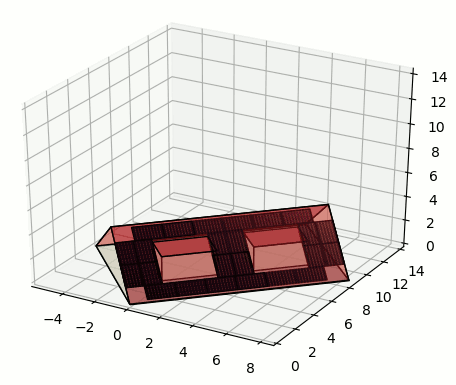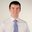Question-and-Answer Resource for the Building Energy Modeling Community
Get started with the Help page

What is the solar irradiance simulation engine for EnergyPlus

May I ask what is the simulation engine for the irradiance / solar related calculation (E.g. the irradiance received by solar panel for the calculation of PV generation) engine that EnergyPlus uses? I checked the documents and seems like it is not using RADIANCE, am I correct?

Possible to couple the use of RADIANCE for E+ simulation (e.g. PV electricity output simulation)?

Thanks

edit retag close merge delete

Sort by » oldest newest most voted

If you want to use the built-in PV models of e+, then using e+'s own irradiance model should be fine to use, because there is a good match between what the e+ irradiance model provides as output (one irradiance value per surface per timestep) and what e+'s PV model requires as input (mean irradiance on the module surface per timestep).

I think it only makes sense to couple Radiance with e+ for calculating irradiance on PV, if the PV system model itself has a higher level of detail than what e+ has (e.g. it is a cell-level model of a PV system like this). Then the approach can be, that you have the e+ geometry, and you can use that to generate the input geometry for the Radiance simulation, which is then used with the high-detail (non-e+) PV simulation to get the power output results. Note, that this is not real coupling of simulations, because after exporting the e+ geometry to Radiance there is no exchange of information between the e+ and irradiance-PV models anymore. They just run in parallel.

If you are interested in this approach, it is still work in progress but you can try Daypym, which is a python library developed to do this. It utilizes Geomeppy for e+ model geometry translation, generates sensor-points and runs DAYSIM (which uses Radiance) irradiance simulations for PV. Se attached output of a simulation for a roof with PV:more

Thank you very much Adam, you gave a very detailed explanation. The reason of asking is that RADIANCE has a lot of documents to show what kind of ray tracing technique they use (backward), the sky model adopted (Perez) etc. while E+ has fewer documents on solar and hence I am not sure whether the simulation engine is as robust as RADIANCE for PV simulation. Thank you for sharing the information of Daypym, seems very useful.Hi,

You are correct.

Have a look on Chapters 5, 6 & 19 of the Engineering Reference.

If you want to see the details of the EnergyPlus simulation, you can download the source and check the files (e.g. Photovoltaics.cc, Photovoltaics.hh, PhotovoltaicThermalCollectors.cc, etc). You can download the files directly from the github repository (../src/EnergyPlus/<'the-files>).

If you want to use Radiance, you can use an External Interface module, OpenStudio, BCVTB, run a script from command-line, etc.

more